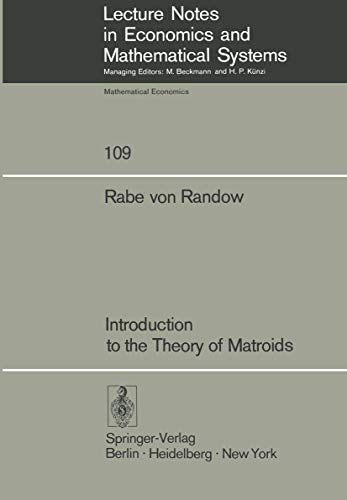Free Shipping in Australia
Proud to be B-Corp

# Introduction to the Theory of Matroids R. von Randow\$164.89
Condition - New
Only 2 left

## Summary

Matroid theory has its origin in a paper by H. Tutte  developed the funda mentals of matroids in detail from a graph-theoretic point of view, and characterised graphic matroids as well as the larger class of those matroids that are representable over any field.

## Introduction to the Theory of Matroids Summary

### Introduction to the Theory of Matroids by R. von Randow

Matroid theory has its origin in a paper by H. Whitney entitled "On the abstract properties of linear dependence" , which appeared in 1935. The main objective of the paper was to establish the essential (abstract) properties of the concepts of linear dependence and independence in vector spaces, and to use these for the axiomatic definition of a new algebraic object, namely the matroid. Furthermore, Whitney showed that these axioms are also abstractions of certain graph-theoretic concepts. This is very much in evidence when one considers the basic concepts making up the structure of a matroid: some reflect their linear algebraic origin, while others reflect their graph-theoretic origin. Whitney also studied a number of important examples of matroids. The next major development was brought about in the forties by R. Rado's matroid generalisation of P. Hall's famous "marriage" theorem. This provided new impulses for transversal theory, in which matroids today play an essential role under the name of "independence structures", cf. the treatise on transversal theory by L. Mirsky [26J. At roughly the same time R.P. Dilworth estab lished the connection between matroids and lattice theory. Thus matroids became an essential part of combinatorial mathematics. About ten years later W.T. Tutte  developed the funda mentals of matroids in detail from a graph-theoretic point of view, and characterised graphic matroids as well as the larger class of those matroids that are representable over any field.

I. Equivalent Axiomatic Definitions and Elementary Properties of Matroids.- 1.1. The first rank-axiomatic definition of a matroid.- 1.2. The independence-axiomatic definition of a matroid.- 1.3. The second rank-axiomatic definition of a matroid.- 1.4. The circuit-axiomatic definition of a matroid.- 1.5. The basis-axiomatic definition of a matroid.- II. Further Properties of Matroids.- 2.1. The span mapping.- 2.2. The span-axiomatic definition of a matroid.- 2.3. Hyperplanes and cocircuits.- 2.4. The dual matroid.- III. Examples.- 3.1. Linear algebraic examples.- 3.2. Binary matroids.- 3.3. Elementary definitions and results from graph theory.- 3.4. Graph-theoretic examples.- 3.5. Combinatorial examples.- IV. Matroids and the Greedy Algorithm.- 4.1. Matroids and the greedy algorithm.- V. Exchange Properties for Bases of Matroids.- 5.1. Symmetric point exchange.- 5.2. Bijective point replacement.- 5.3. More on minors of a matroid.- 5.4. Symmetric set exchange.- 5.5. Bijective set replacement.- 5.6. A further symmetric set exchange property.

NLS9783540071778
9783540071778
3540071776
Introduction to the Theory of Matroids by R. von Randow
New
Paperback
Springer-Verlag Berlin and Heidelberg GmbH & Co. KG
1975-06-23
106
N/A
Book picture is for illustrative purposes only, actual binding, cover or edition may vary.
This is a new book - be the first to read this copy. With untouched pages and a perfect binding, your brand new copy is ready to be opened for the first time